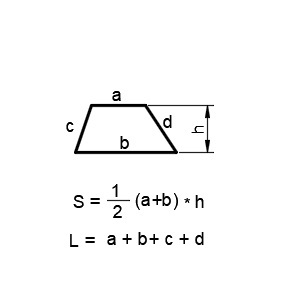# Calculating the trapezoid area

On this page, the calculator will help you calculate the area of the trapezoid online. To calculate, set the height and length of the trapezoid base.

A trapezoid is a quadrilateral whose two sides are parallel (the bases of the trapezoid) and the other two are non - parallel (the sides of the trapezoid). The segment connecting the midpoints of the sides is called the midline of the trapezoid.

 Top of the trapezoid a Base trapézoïdale b The altitude of the trapezoid h Area of a trapezoid